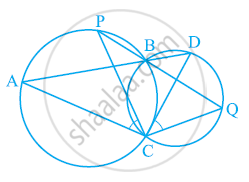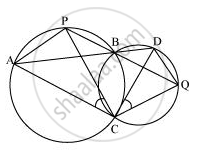# Two circles intersect at two points B and C. Through B, two line segments ABD and PBQ are drawn to intersect the circles at A, D and P, Q respectively (see the given figure). Prove that ∠ACP = ∠QCD. - Mathematics

Two circles intersect at two points B and C. Through B, two line segments ABD and PBQ are drawn to intersect the circles at A, D and P, Q respectively (see the given figure). Prove that ∠ACP = ∠QCD.#### SolutionJoin chords AP and DQ.

For chord AP,

∠PBA = ∠ACP (Angles in the same segment) ... (1)

For chord DQ,

∠DBQ = ∠QCD (Angles in the same segment) ... (2)

ABD and PBQ are line segments intersecting at B.

∴ ∠PBA = ∠DBQ (Vertically opposite angles) ... (3)

From equations (1), (2), and (3), we obtain

∠ACP = ∠QCD

Is there an error in this question or solution?

#### APPEARS IN

NCERT Class 9 Maths
Chapter 10 Circles
Exercise 10.5 | Q 9 | Page 186